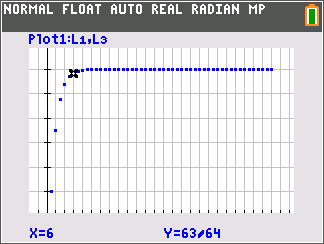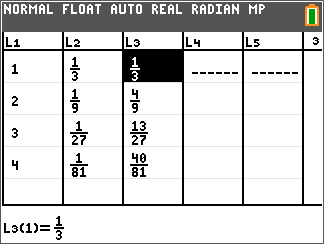# QLD (TI-84PlusCE): Summing Up Geometric Series

by Texas Instruments#### Overview

In this activity, students will explore infinite geometric series and the partial sums of geometric series. The students will determine the limits of these sequences and series using tables and graphs.

#### Key Steps

•Students will find a partial sum of two geometric series.

•Students will graph the series by generating a list with the cumulative sums of the terms of the sequence.

•Students will find the sum of a geometric series by taking the limit of the nth sum.